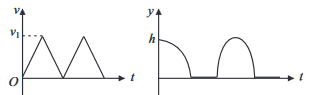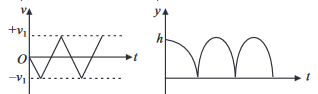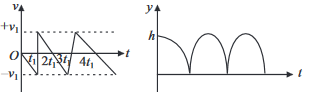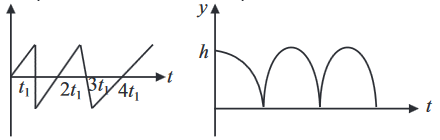#### Consider a rubber ball freely falling from a heightonto a horizontal elastic plate. Assume that the duration of collision is negligible and the collision with the plate is totally elastic. Then the velocity as a function of time and the height as function of time will be Option 1)Option 2)Option 3)Option 4)As we learnt in

If only conservative forces act on a system, total mechnical energy remains constant --

As the ball fall its velocity increasesas it reflect after collistionSo velocity is linear.

Increasing while going down and decreasing while going up.So correct graph is 3

Option 1)This is an incorrect option.

Option 2)This is an incorrect option.

Option 3)This is the correct option.

Option 4)This is an incorrect option.

##### Posted by